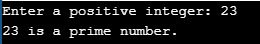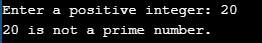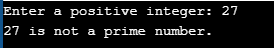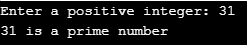# Types of User Defined Functions in C

## Types of User-Defined Functions in C Language

In this article, I will discuss the Types of User-Defined Functions in C Language with examples. Please read our previous articles discussing the Functions in C Language with Examples.

##### Types of User-Defined Functions in C Language:

In C programming, user-defined functions are a fundamental component that allows programmers to break down complex problems into smaller, manageable parts. Users write these functions to perform specific tasks and can be categorized based on their return type and the arguments they accept. There are four types of user-defined functions in C. They are as follows:

1. Functions with No Argument and No Return Type.
2. Functions with Argument and No Return Type.
3. Functions with No Argument and with Return Type.
4. Functions with Argument and with Return Type

Let us understand each of these function types with examples.

##### Functions with No Argument and No Return Value in C Language:

This type of function neither accepts any input nor returns any value. It’s often used for performing a specific task, such as displaying a message.

Example: A function to display a welcome message.

```#include <stdio.h>

void displayMessage() {
printf("Hello, this is a simple message.\n");
}

int main() {
displayMessage();
return 0;
}```
###### Key Points:
• These functions do not take any arguments and do not return a value.
• They are used to perform a specific task that does not require any input and does not need to provide any output.
###### Program to Understand No Arguments Passed and No Return Value Function in C Language:
```#include <stdio.h>
int main()
{
checkPrimeNumber();    // argument is not passed
return 0;
}

// return type is void meaning doesn't return any value
{
int n, i, flag = 0;
printf("Enter a positive integer: ");
scanf("%d",&n);
for(i=2; i <= n/2; ++i)
{
if(n%i == 0)
{
flag = 1;
}
}
if (flag == 1)
printf("%d is not a prime number.", n);
else
printf("%d is a prime number.", n);
}```
###### Output:The checkPrimeNumber() function takes input from the user, checks whether it is a prime number, and displays it on the screen. The empty parentheses in checkPrimeNumber(); statement inside the main() function indicates that no argument is passed to the function. The return type of the function is void. Hence, no value is returned from the function.

##### Functions with Arguments but No Return Value in C Language:

These functions take input parameters but do not return a value. They perform operations on the inputs but don’t provide any output.
Example: A function to print the sum of two numbers passed as arguments.

```#include <stdio.h>

void printSum(int a, int b) {
int sum = a + b;
printf("Sum is: %d\n", sum);
}

int main() {
printSum(5, 7);
return 0;
}
```
###### Key Points:
• These functions take one or more arguments but do not return any value.
• They are used for operations where input is required, but the result of the operation does not need to be returned.
##### Program to Understand No Arguments Passed but Return a Value Function in C Language
```#include <stdio.h>
int getInteger();
int main()
{
int n, i, flag = 0;
// no argument is passed
n = getInteger();
for(i=2; i<=n/2; ++i)
{
if(n%i==0){
flag = 1;
break;
}
}
if (flag == 1)
printf("%d is not a prime number.", n);
else
printf("%d is a prime number.", n);
return 0;
}
// returns integer entered by the user
int getInteger()
{
int n;
printf("Enter a positive integer: ");
scanf("%d",&n);
return n;
}```
###### Output:The empty parentheses in the n = getInteger(); statement indicate that no argument is passed to the function. The value returned from the function is assigned to n. Here, the getInteger() function takes input from the user and returns it. The code to check whether a number is prime or not is inside the main() function.

##### Functions with No Argument but with a Return Value in C Language:

Such functions do not take any input but return a value. They’re useful when a result is required without input.
Example: A function that returns a random number.

```#include <stdio.h>

int getRandomNumber() {
return rand() % 100;  // Returns a random number between 0 and 99
}

int main() {
printf("Random number: %d\n", getRandomNumber());
return 0;
}
```
###### Key Points:
• These functions do not take any arguments but return a value.
• It is useful in scenarios where a function needs to perform an operation and return the result, but no input is required from the user.
###### Program to Understand Argument Passed but no Return Value Function in C Language
```#include <stdio.h>
void checkPrimeAndDisplay(int n);
int main()
{
int n;
printf("Enter a positive integer: ");
scanf("%d",&n);
// n is passed to the function
checkPrimeAndDisplay(n);
return 0;
}
// return type is void meaning doesn't return any value
void checkPrimeAndDisplay(int n)
{
int i, flag = 0;
for(i=2; i <= n/2; ++i)
{
if(n%i == 0){
flag = 1;
break;
}
}
if(flag == 1)
printf("%d is not a prime number.",n);
else
printf("%d is a prime number.", n);
}```
###### Output:The integer value the user enters is passed to the checkPrimeAndDisplay() function. Here, the checkPrimeAndDisplay() function checks whether the argument passed is a prime number and displays the appropriate message.

##### Functions with Arguments and a Return Value in C Language:

These are versatile functions that take input parameters and also return a value.
Example: A function that takes two numbers as arguments and returns their multiplication.

```#include <stdio.h>

int multiply(int a, int b) {
return a * b;
}

int main() {
int result = multiply(4, 5);
printf("Product is: %d\n", result);
return 0;
}```
###### Key Points:
• These functions take one or more arguments and also return a value.
• They are the most flexible type of function, allowing for input and output.
###### Program to Understand Argument Passed and Return Value Function in C Language
```#include <stdio.h>
int main()
{
int n, flag;
printf("Enter a positive integer: ");
scanf("%d",&n);
// n is passed to the checkPrimeNumber() function
// the returned value is assigned to the flag variable
if(flag == 1)
printf("%d is not a prime number",n);
else
printf("%d is a prime number",n);
return 0;
}
// int is returned from the function
{
int i;
for(i=2; i <= n/2; ++i)
{
if(n%i == 0)
return 1;
}
return 0;
}```
###### Output:The input from the user is passed to the checkPrimeNumber() function. The checkPrimeNumber() function checks whether the passed argument is prime. If the passed argument is a prime number, the function returns 0. If the passed argument is a non-prime number, the function returns 1. The return value is assigned to the flag variable. Depending on whether the flag is 0 or 1, an appropriate message is printed from the main() function.

##### Do you know how many values can be returned from C functions?

Always, only one value can be returned from a function. If you try to return more than one value from a function, only one value that appears at the rightmost place of the return statement will be returned.

For example, if you use “return a,b,c” in your function, the value for c only will be returned, and values a and b won’t be returned to the program. If you want to return more than one value, pointers can change the values in the address directly instead of returning those values to the function.